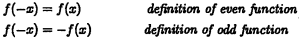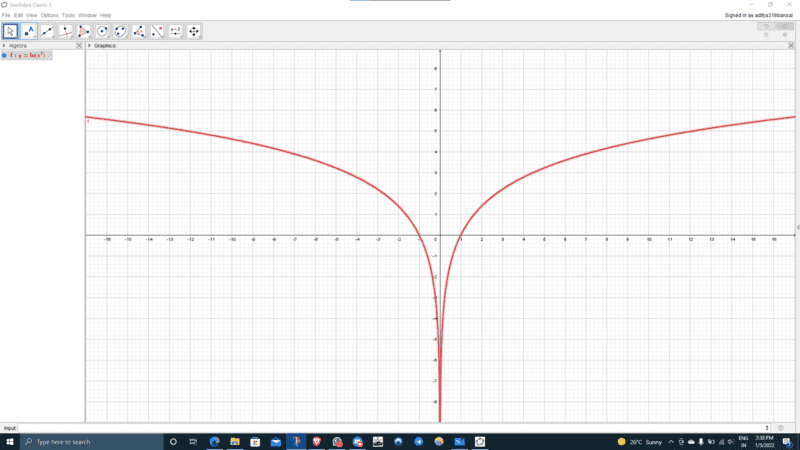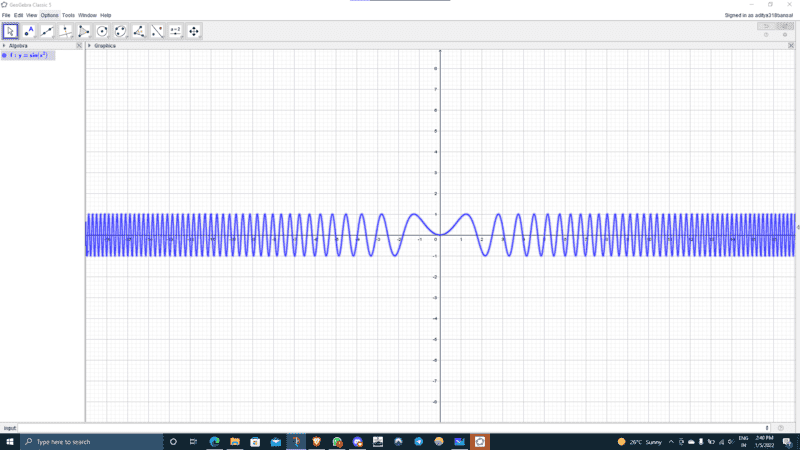# The given function is an even or an odd function?

brochesspro
Homework Statement:
Determine whether the function ##f(x^2)## is an even function, or an odd function, or neither, where ##f(x)## is an arbitrary function.
Relevant Equations:
Given below.I think the answer is an even function as the function ##x^2## is an even function and thus, is symmetrical w.r.t. Y axis. The question I have is how to do this problem algebraically. I tried to graph some functions on GeoGebra to verify my answer.

a) ##y = ln(x^2)##b) ##y = sin(x^2)##These are the two functions I tried, and both look pretty even to me. Please help me solve this problem. Thank you.

•Delta2

Homework Helper
Gold Member
2022 Award
How is the function ##f(x^2)## defined?

Homework Helper
Gold Member
2022 Award
PS how did the computer calculate the function values to draw that graph for you?

brochesspro
PS how did the computer calculate the function values to draw that graph for you?

The computer first calculates the values of the squares of the input value and applies the arbitrary function to the new value. I know that since the function ##x^2## is an even function and thus, is symmetrical w.r.t. Y axis, the output of the arbitrary function will be even. Eg. ##f((-1)^2) = f(1^2)## as ##(-1)^2 = 1^2 = 1##. I am sure I am correct till here. Are you?

I will see you after two hours.

Last edited:
Homework Helper
Gold Member
2022 Award
The computer first calculates the values of the squares of the input value and applies the arbitrary function to the new value.
Exactly. This is called the composition of two functions. Technically, we define a new function ##g(x)## by:$$g(x) = f(x^2)$$And then ##g(x)## is an even function for any function ##f(x)##, as you have shown for ##x = 1##.

brochesspro
Exactly. This is called the composition of two functions. Technically, we define a new function ##g(x)## by:$$g(x) = f(x^2)$$And then ##g(x)## is an even function for any function ##f(x)##, as you have shown for ##x = 1##.
But how do I write it formally?

Homework Helper
Gold Member
You should directly use the definitions of "even" and "odd" functions. Start with ##g(-x) = f((-x)^2)## and see where that leads you for the function g. Is ##g(x)## even or odd or neither?

Mentor
But how do I write it formally?
##f((-x)^2) = f(x^2)##, for ##x^2## in the domain of f.
Thus ##f(x^2)## is an even function.

••sysprog and Delta2
brochesspro
##f((-x)^2) = f(x^2)##, for ##x^2## in the domain of f.
Thus ##f(x^2)## is an even function.
You should directly use the definitions of "even" and "odd" functions. Start with ##g(-x) = f((-x)^2)## and see where that leads you for the function g. Is ##g(x)## even or odd or neither?
Oh, I see. Thank you.

Homework Helper
Gold Member
To do it fully formally you have first to define the function $$g(x)=f(x^2)$$ and then prove that $$g(-x)=g(x)$$ and hence g is even. The proof is half a line long, it is essentially what @Mark44 does at post #8.

To do it fully formally you have first to define the function $$g(x)=f(x^2)$$ and then prove that $$g(-x)=g(x)$$ and hence g is even. The proof is half a line long, it is essentially what @Mark44 does at post #8.
••